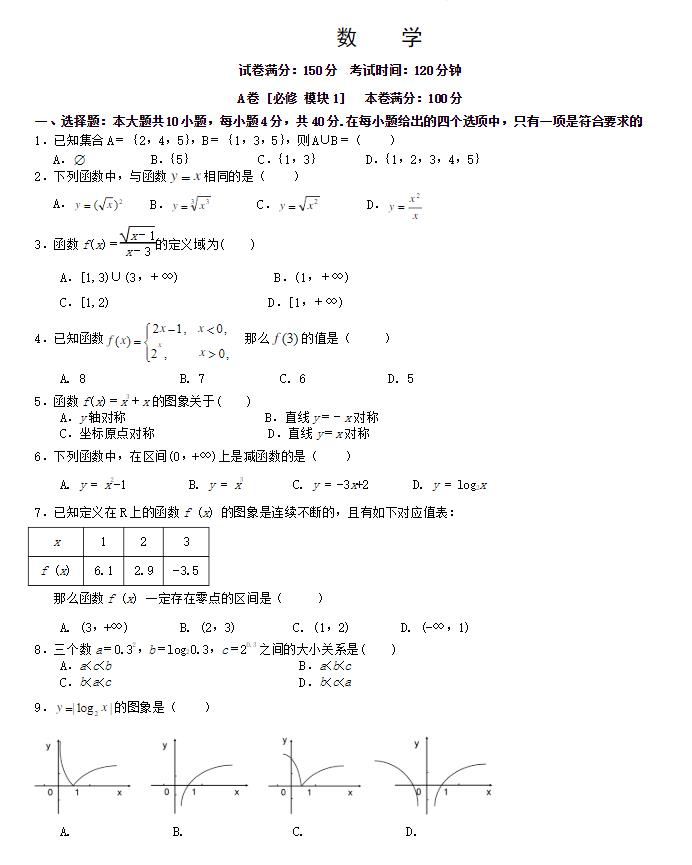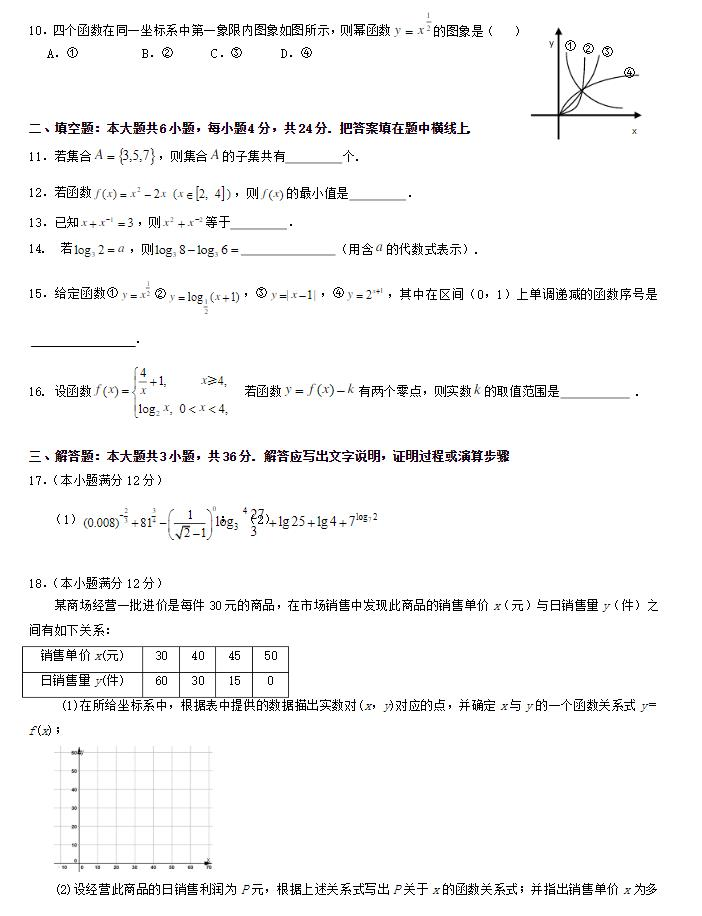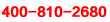<返回切换城市

## 北京高一期中考试数学

2020-04-08 14:57:00 来源：佚名北京高一期中考试数学高中数学函数知识点

1.函数的奇偶性

(1)若f(x)是偶函数，那么f(x)=f(-x);

(2)若f(x)是奇函数，0在其定义域内，则f(0)=0(可用于求参数);

(3)判断函数奇偶性可用定义的等价形式：f(x)±f(-x)=0或(f(x)≠0);

(4)若所给函数的解析式较为复杂，应先化简，再判断其奇偶性;

(5)奇函数在对称的单调区间内有相同的单调性;偶函数在对称的单调区间内有相反的单调性;

2.复合函数

(1)复合函数定义域求法：若已知的定义域为[a，b],其复合函数f[g(x)]的定义域由不等式a≤g(x)≤b解出即可;若已知f[g(x)]的定义域为[a,b],求f(x)的定义域，相当于x∈[a,b]时，求g(x)的值域(即f(x)的定义域);研究函数的问题一定要注意定义域优先的原则。

(2)复合函数的单调性由“同增异减”判定;

3.函数图像(或方程曲线的对称性)

(1)证明函数图像的对称性，即证明图像上任意点关于对称中心(对称轴)的对称点仍在图像上;

(2)证明图像C1与C2的对称性，即证明C1上任意点关于对称中心(对称轴)的对称点仍在C2上，反之亦然;

(3)曲线C1：f(x,y)=0,关于y=x+a(y=-x+a)的对称曲线C2的方程为f(y-a,x+a)=0(或f(-y+a,-x+a)=0);

(4)曲线C1:f(x,y)=0关于点(a,b)的对称曲线C2方程为：f(2a-x,2b-y)=0;

(5)若函数y=f(x)对x∈R时，f(a+x)=f(a-x)恒成立，则y=f(x)图像关于直线x=a对称;

(6)函数y=f(x-a)与y=f(b-x)的图像关于直线x=对称;

点击查看：高中数学知识点总结

获取完整版方法：

1.点击链接：https://jinshuju.net/f/1Ki3F0 领取2014-2019北京中考真题、北京各区一模、二模试卷及答案解析汇总，填写姓名、电话信息后即可跳转百度云盘地址，免费获取。

这一期的北京高一期中考试数学小编就介绍到这里，高中数学与初中数学是完全不同的两个概念，最大的区别就是，高中数学更加抽象了想了解相关课程的同学，请拨打学而思爱智康免费咨询电话：小编推荐：北京中考数学第十题相关课程推荐相关文章推荐